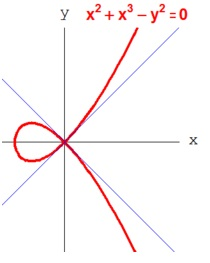#### Algebric Geometry Homework Help - K-12 Grade Level, College Level Mathematics

Introduction to Algebric Geometry

The algebric geometry is a category of mathematics. Algebric geometry is the study on properties of shapes defined by zeros of polynomial equations. The modern algebraic geometry is totally based on many more abstract methods of abstract algebra particularly commutative algebra with the language and the problems of geometry.The fundamental objects of study in algebric geometry are algebric varieties that are geometric manifestations of solutions of systems of polynomial equations. The illustrations of algebric varieties are: a plane algebraic curve which involves circles, lines, parabolas, hyperbolas, ellipses, cubic curves like elliptic curves and quartic curves like lemniscates, and Cassini ovals. Points of the plane belong to an algebraic curve if its coordinates satisfy a given polynomial equation. The basic questions which involve the study of the points of special interest like the inflection points, singular points and points at infinity. More advance questions includes the topology of the curve and relations among the curves given by various equations.

The algebraic geometry inhabits a central place in modern mathematics and has multiple conceptual connections with these diverse fields as complex analysis, number theory and topology. Primarily a study of systems of polynomial equations in different variables, the subject of algebraic geometry begins where equation solving leaves off and it becomes more significant to understand the intrinsic properties of the totality of solutions of a system of equations, than to find a solution which is specific; this leads into some of the deepest regions in all of mathematics both conceptually and in terms of method.

In 20th century the algebric geometry has subdivided into various subareas.

The main stream of algebraic geometry is dedicated to the study of the complex points of the algebraic ranges and usually to the points with coordinates in an algebraically closed field. The study of real points of an algebraic range is the subject of real algebraic geometry. The study of points of an algebraic range with coordinates in the field of the rational numbers or in a number field became arithmetic geometry (more typically Diophantine geometry) a subfield of the algebraic number theory.

A very big part of the theory of singularity is dedicated to the singularities of the algebraic varieties.

With the rise of the computers, a computational algebraic geometry region has emerged which lies at the intersection of algebraic geometry and computer algebra. It consists necessarily developing software and algorithms for studying and finding the properties of explicitly given algebraic varieties.

Real algebric geometry

It is the study of real points of the algebric geometry.

The fact that the field of the real number is an ordered field might not be occulted in such a study. For illustration, the curve of equation x2 + y2 - a = 0 is a circle when a>0, but does not have any real point if a<0. It pursue that the real algebraic geometry is not only the study of the real algebraic varieties however has been generalized to the study of the semi-algebraic sets which are the solutions of systems of polynomial equations and polynomial inequalities. For illustration, a branch of the hyperbola of equation xy - 1 = 0 is not an algebraic range but is a semi-algebraic set defined by xy - 1 = 0 and x>0 or xy - 1 = 0 by and x+y>0.

One of the challenging problems of real algebraic geometry is unsolved Hilbert's sixteenth problem: Decide which respective positions are possible for the ovals of a non-singular plane curve of degree 8.

Email based Algebric geometry Homework Help -Assignment Help

Tutors at the www.tutorsglobe.com are committed to provide the best quality Algebric geometry homework help - assignment help. They use their experience, as they have solved thousands of the Algebric geometry assignments, which may help you to solve your complex Algebric geometry homework. You can find solutions for all the topics come under the Algebric geometry. The dedicated tutors provide eminence work on your Math homework help and devoted to provide K-12 level Math to college level Math help before the deadline mentioned by the student. Algebraic geometry homework help is available here for the students of school, college and university. TutorsGlobe assure for the best quality compliance to your homework. Compromise with quality is not in our dictionary. If we feel that we are not able to provide the homework help as per the deadline or given instruction by the student, we refund the money of the student without any delay.

Qualified and Experienced Algebric geometry Tutors at www.tutorsglobe.com

Tutors at the www.tutorsglobe.com take pledge to provide full satisfaction and assurance in Algebric geometry homework help. Students are getting Math homework help services across the globe with 100% satisfaction. We value all our service-users. We provide email based Algebric geometry homework help - assignment help. You can join us to ask queries 24x7 with live, experienced and qualified Math tutors specialized in Algebric geometry.